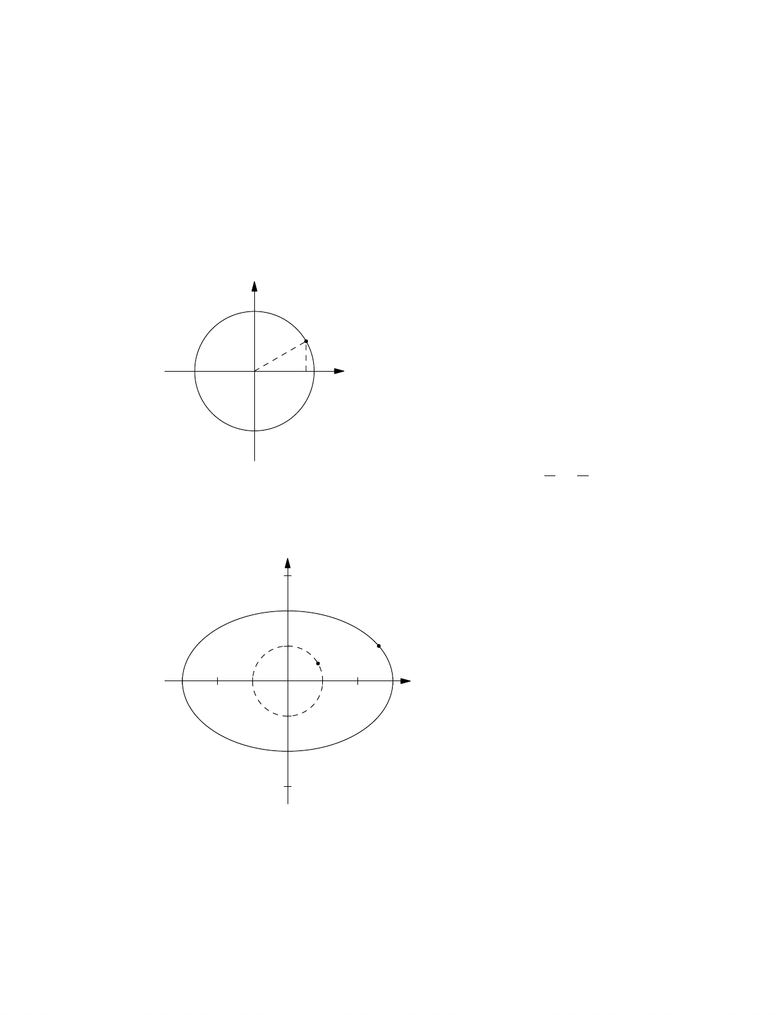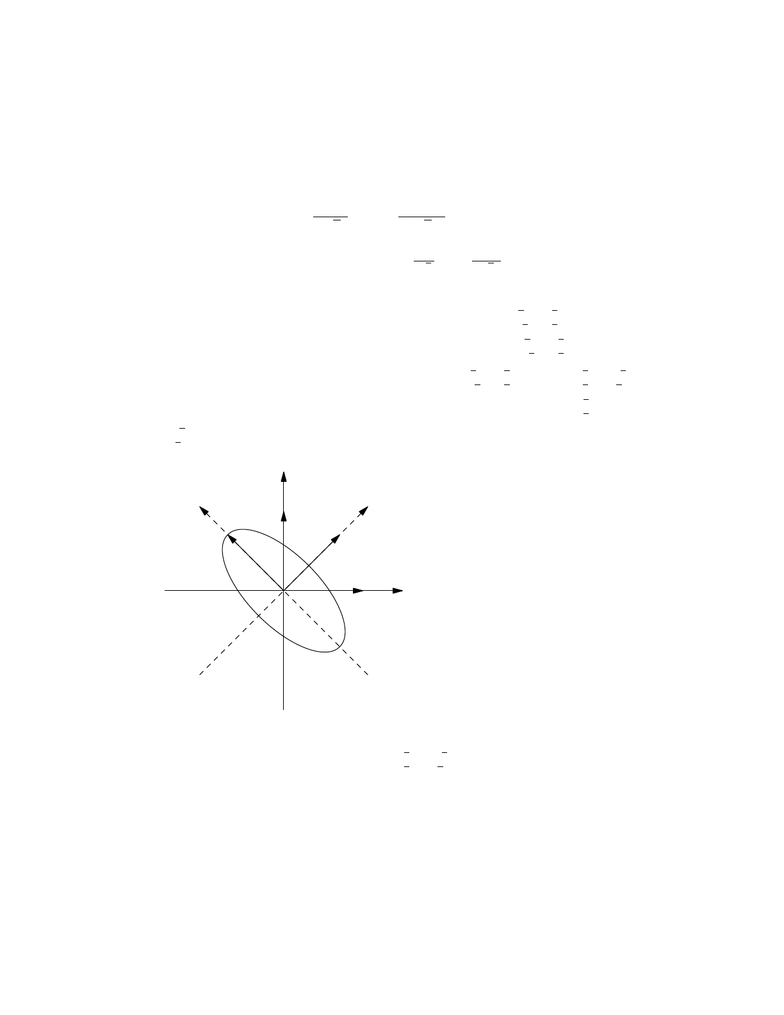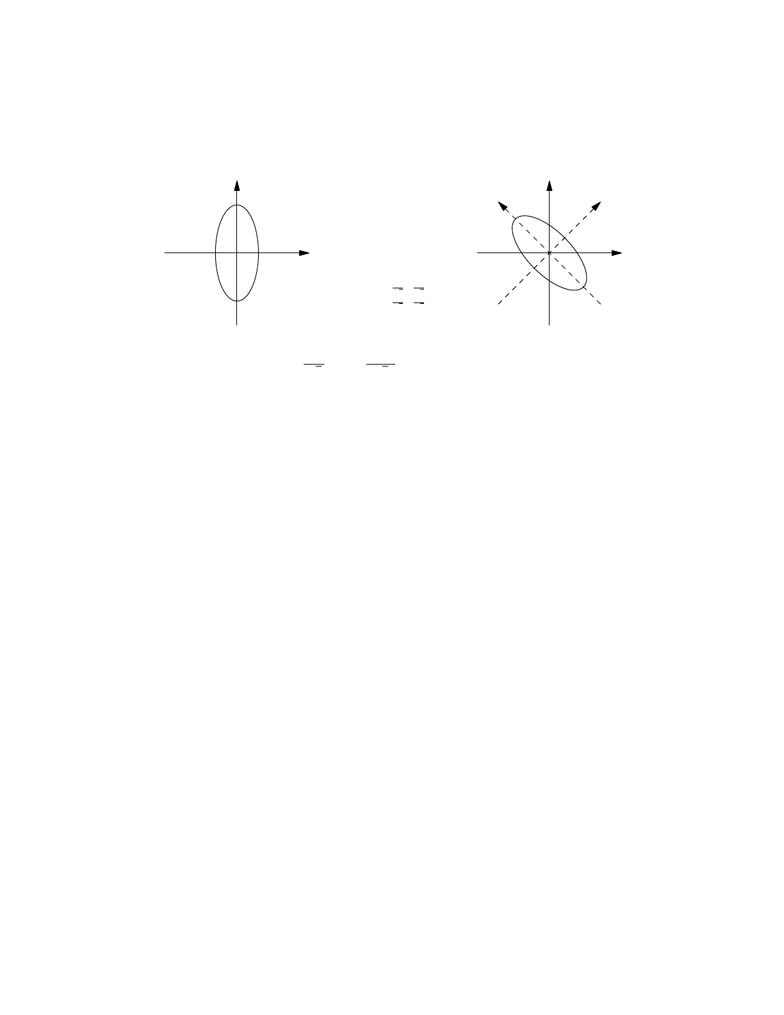# Drawing planar quadratic, quadratic forms, orthonormal basis and matricies, gram-schmidt orthogonalization process

33 views8 pages
School
UTSG
Department
Mathematics
Course
MAT224H1
ProfessorLecture 9notes byY. Burda
Weall know what the equation x2+y2=1describes: it is the equation of a
unit circle:
(x, y)
1
It is easy to see what shapeis described bythe equation x2
9+y2
4=1. If
apoint (x, y)lies onthis shape, then the point (x/3,y/2) lies on the unit
circle. So in fact what wehaveis the result of stretching the unit circle bya
factor of 3in the xdirection and byafactor of 2in ydirection. The resulting
shapeis called an ellipse.
(x, y)
(x/3,y/2)
Muchharder is to describehowthe locus of points (x, y)satisfying 3x2+
4xy +3y2=1looks like. In fact wewill spend the next twolectures learning
1
www.notesolution.com
Unlock document

This preview shows pages 1-3 of the document.
Unlock all 8 pages and 3 million more documents.howto bring this equation to the muchmore manageable form
5x+y
22
+x+y
22
=1
For this equation the coordinates ˜x=x+y
2,˜y=x+y
2seem to beuseful, as
in these coordinates the equationhas the familiar form 5˜x2+˜y2=1, which
describes anellipse.
What is the meaning of these coordinates ˜x
˜y=1/2 1/2
1/2 1/2(x
y)? These
are the coordinates relativeto abasis Bwith [I]B,E=1/2 1/2
1/2 1/2.The vec-
tors from Bare then the columns of [I]E,B=1/2 1/2
1/2 1/21=1/21/2
1/2 1/2.
Thus ˜x, ˜yare the coordinates relativeto the basis v1=1/2
1/2,v2=
1/2
1/2.Now wecan drawour ellipse:
x
y
e1
e2
˜x
˜y
v1
v2
Alternatively wecould havedrawn the ellipse in the plane with coordi-
nates ˜x, ˜yand then the shapeweare after would beits image underthe linear
transformation sending ˜x
˜yto (x
y)=1/21/2
1/2 1/2(x
y). One can verify that this
is the transformation of rotation by45in counterclockwise direction, so we
2
www.notesolution.com
Unlock document

This preview shows pages 1-3 of the document.
Unlock all 8 pages and 3 million more documents.˜x
˜y
˜x
˜y
x
y
˜x
˜y! x
y!= 1
21
2
1
2
1
2! ˜x
˜y!
Wewill nowtry to attackthe questions “howto go from the form 3x2+
4xy +3y2to the form 5x+y
22+x+y
22?”, “when drawing in anew system
of coordinates is as easy as drawing in the usual coordinates?” and “howcan
weuse what weknowfrom linearalgebra tohelp us with these questions?”.
The expression 3x2+4xy +3y2is an example of aquadratic form in variables
x, y.Other examples include the forms xy and x2+5y2.
Wecan also form quadratic formsin three variables, for instance x2+
2y2+3z2+5xy +7xz +9yz.
The general deﬁnition is the following:
Deﬁnition. Areal quadratic form in nvariables is afunction q:RnR
satisfying
q(v)=X
1in
1jn
cijxixj
wherecij aresome ﬁxedreal numbers and x1,...,xnare coordinates of v
relative to the standardbasis of Rn.
Wecan put the coeﬃcients cij from this deﬁnition into an n×nmatrix.
It will turn outto bevery convenientto us in the future. For instance we
can rewrite 3x2+4xy +5y2as
x·(3x+4y)+y·(5y)=(xy)3x+4y
5y=(xy)(3 4
0 5) (x
y)
The coeﬃcients in the matrix (3 4
0 5)are in fact the coeﬃcients in the form
3x2+4xy +5y2westarted with: 3is the coeﬃcientat x2,5isthe coeﬃcient
at y2,4is the coeﬃcientat xy.
3
www.notesolution.com
Unlock document

This preview shows pages 1-3 of the document.
Unlock all 8 pages and 3 million more documents.